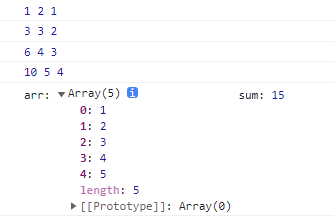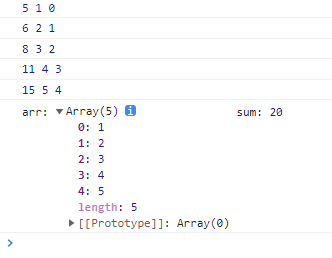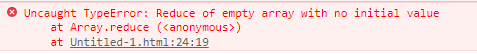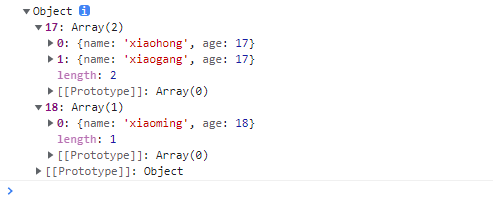0

# JS 中 reduce()方法及使用详解

reduce()方法可以搞定的东西特别多，就是循环遍历能做的，reduce都可以做，比如数组求和、数组求积、统计数组中元素出现的次数、数组去重等等。

reduce() 方法对数组中的每个元素执行一个由您提供的reduce函数(依次执行)，将其结果汇总为单个返回值。

## 1、语法介绍

``````// arr.reduce(callback,[initialValue])

array.reduce((prev, cur, index, arr)=> {
/***/
}, initialValue)
``````

reduce 为数组中的每一个元素依次执行回调函数，接受四个参数：初始值 initialValue（或者上一次回调函数的返回值），当前元素值，当前索引，调用 reduce 的数组。

### 参数：

** 参数一： callback 函数（执行数组中每个值的函数，包含四个参数）**

• ``````prev 必需 （上一次调用回调返回的值，或者是提供的初始值（initialValue））
``````
• ``````cur 必需（数组中当前被处理的元素）
``````
• ``````index 可选 （当前元素在数组中的索引）
``````
• ``````arr 可选 （调用 reduce 的数组）
``````

``````    **提供初始值**，cur 从数组**第一项**开始，若**不提供初始值**，则 cur 从**第二项**开始执行，对应的第一次 prev 是数组**第一项**的值
``````

## 2、实例解析 initialValue 参数

### 示例一：

• 没有设置函数的初始迭代值
``````<script>
let arr = [1, 2, 3, 4, 5];
let sum = arr.reduce(function (prev, cur, index, arr) {
console.log(prev, cur, index);
return prev + cur;
});
console.log('arr:', arr, 'sum:', sum);
</script>
``````** 分析：在这里reduce的作用就是对这个数组进行求和，这里可以看出，数组长度是5，但是reduce函数循环4次，函数迭代的初始值**是1，也就是默认值（数组的第一项），index是从1开始的，第一次的prev的值是数组的第一个值，而之后prev的值是每次计算后的值。

### 再看示例二：

• 设置函数的初始迭代值
``````<script>
let arr = [1, 2, 3, 4, 5];
let sum = arr.reduce(function (prev, cur, index, arr) {
console.log(prev, cur, index);
return prev + cur;
}, 5) //注意这里设置了初始值
console.log("arr:", arr, "sum:", sum);
</script>
``````## 3、注意事项

1. reduce是一个对数组累积操作的方法，使用时要加上return返回累积操作的数据。这样 prev 才能获取上一次执行的结果，否则是 undefined；

2. 空数组执行 reduce 操作且不提供初始值时reduce会报错，错误信息如下：``````<script>
// 空数组的情况
let arr = [];
let sum = arr.reduce(function (prev, cur, index, arr) {
console.log(prev, cur, index);
return prev + cur;
})
//报错，"TypeError: Reduce of empty array with no initial value"
</script>
``````

``````<script>
// 空数组，但设置 初始值 的情况
let arr = [];
let sum = arr.reduce(function (prev, cur, index, arr) {
console.log(prev, cur, index);
return prev + cur;
}, 0)
console.log(arr, sum); // [] 0
</script>
``````

## 4、reduce的应用

### ** （1）、最简单的就是我们常用的 数组求和，求乘积了。**

``````let arr = [1, 2, 3, 4];
let sum = arr.reduce((x,y)=>x+y)
let mul = arr.reduce((x,y)=>x*y)
console.log( sum ); //求和，10
console.log( mul ); //求乘积，24
``````

### ** （2）、计算数组中每个元素出现的次数**

``````<script>
let names = ['Alice', 'Bob', 'Tiff', 'Bruce', 'Alice'];
let nameNum = names.reduce((pre, cur) => {
if (cur in pre) {
pre[cur]++
} else {
pre[cur] = 1
}
return pre
}, {})
console.log(nameNum); //{Alice: 2, Bob: 1, Bruce: 1, Tiff: 1}
</script>
``````

1. 由于设置了迭代初始值，pre的第一个值是一个空对象，此时name为Alice，然后进行判断，发现在pre中没有Alice属性，所以就将Alice对应的属性值赋为1。
2. 后面没有重复的是一样的道理，如果碰到重复值，就会将该属性值加1，这样就能计算元素重复的次数了。

### ** （3）、数组去重**

``````<script>
let arr = [1, 2, 3, 4, 4, 1]
let newArr = arr.reduce((pre, cur) => {
if (!pre.includes(cur)) {
return pre.concat(cur)
} else {
return pre
}
}, [])
console.log(newArr);// [1, 2, 3, 4]
</script>
``````

### （4）、将二维数组转化为一维

``````<script>
let arr = [[0, 1], [2, 3], [4, 5]]
let newArr = arr.reduce((pre, cur) => {
return pre.concat(cur)
}, [])
console.log(newArr); // [0, 1, 2, 3, 4, 5]
</script>
``````

### （5）、将多维数组转化为一维

``````<script>
let arr = [[0, 1], [2, 3], [4, [5, 6, 7]]]
const newArr = function (arr) {
return arr.reduce((pre, cur) => pre.concat(Array.isArray(cur) ? newArr(cur) : cur), [])
}
console.log(newArr(arr)); //[0, 1, 2, 3, 4, 5, 6, 7]
</script>
``````

### （6）、对象里的属性求和

``````<script>
let result = [
{
subject: 'math',
score: 10
},
{
subject: 'chinese',
score: 20
},
{
subject: 'english',
score: 30
}
];

var sum = result.reduce(function (prev, cur) {
return cur.score + prev;
}, 0);
console.log(sum) //60
</script>
``````

### （6）、按属性对Object分类

``````let person = [
{
name: 'xiaoming',
age: 18
},{
name: 'xiaohong',
age: 17
},{
name: 'xiaogang',
age: 17
}
];
function groupBy(objectArray, property) {
return objectArray.reduce(function (acc, obj) {
var key = obj[property];
if (!acc[key]) {
acc[key] = [];
}
acc[key].push(obj);
return acc;
}, {});
}
let groupedPerson = groupBy(person, 'age');
console.log(groupedPerson);
``````## 总结：

reduce() 是数组的归并方法，与 forEach()、map()、filter()等迭代方法一样都会对数组每一项进行遍历，但是reduce() 可同时将前面数组项遍历产生的结果与当前遍历项进行运算，这一点是其他迭代方法无法企及的。

### “JS 中 reduce()方法及使用详解”的评论:

##### 关于作者##### overfit同步小助手